Jarvis' Blog (总有美丽的风景让人流连) 总有美丽的风景让人流连

# Prompt 学习和微调 (Prompt Learning and Tuning)

2022-04-20
Jarvis
Post

Self-Attention 和 Transformer 自从问世就成为了自然语言处理领域的新星. 得益于全局的注意力机制和并行化的训练, 基于 Transformer 的自然语言模型能够方便的编码长距离依赖关系, 同时在大规模自然语言数据集上并行训练成为可能. 但由于自然语言任务种类繁多, 且任务之间的差别不太大, 所以为每个任务单独微调一份大模型很不划算. 在 CV 中, 不同的图像识别任务往往也需要微调整个大模型, 也显得不够经济. Prompt Learning 的提出给这个问题提供了一个很好的方向.

## 2. Prompt Learning

### 2.1 什么是 Prompt ?

• 情感预测任务. 输入: “I missed the bus today. I felt so ___.” 其中 “I felt so” 就是提示词 (prompt), 然后使用 LM 用一个表示情感的词填空.
• 翻译任务. 输入: “English: I missed the bus today. French: ___.” 其中 “English:” 和 “French:” 就是提示词, 然后使用 LM 应该再空位填入相应的法语句子.

### 2.2 有哪些预训练模型?

• Left-to-Right LM: GPT, GPT-2, GPT-3
• Prefix LM: UniLM1, UniLM2
• Encoder-Decoder: T5, MASS, BART

### 2.3 有哪些 Prompt Learning 的方法?

• 按照 prompt 的形状划分: 完形填空式, 前缀式.
• 按照人的参与与否: 人工设计的, 自动的(离散的, 连续的)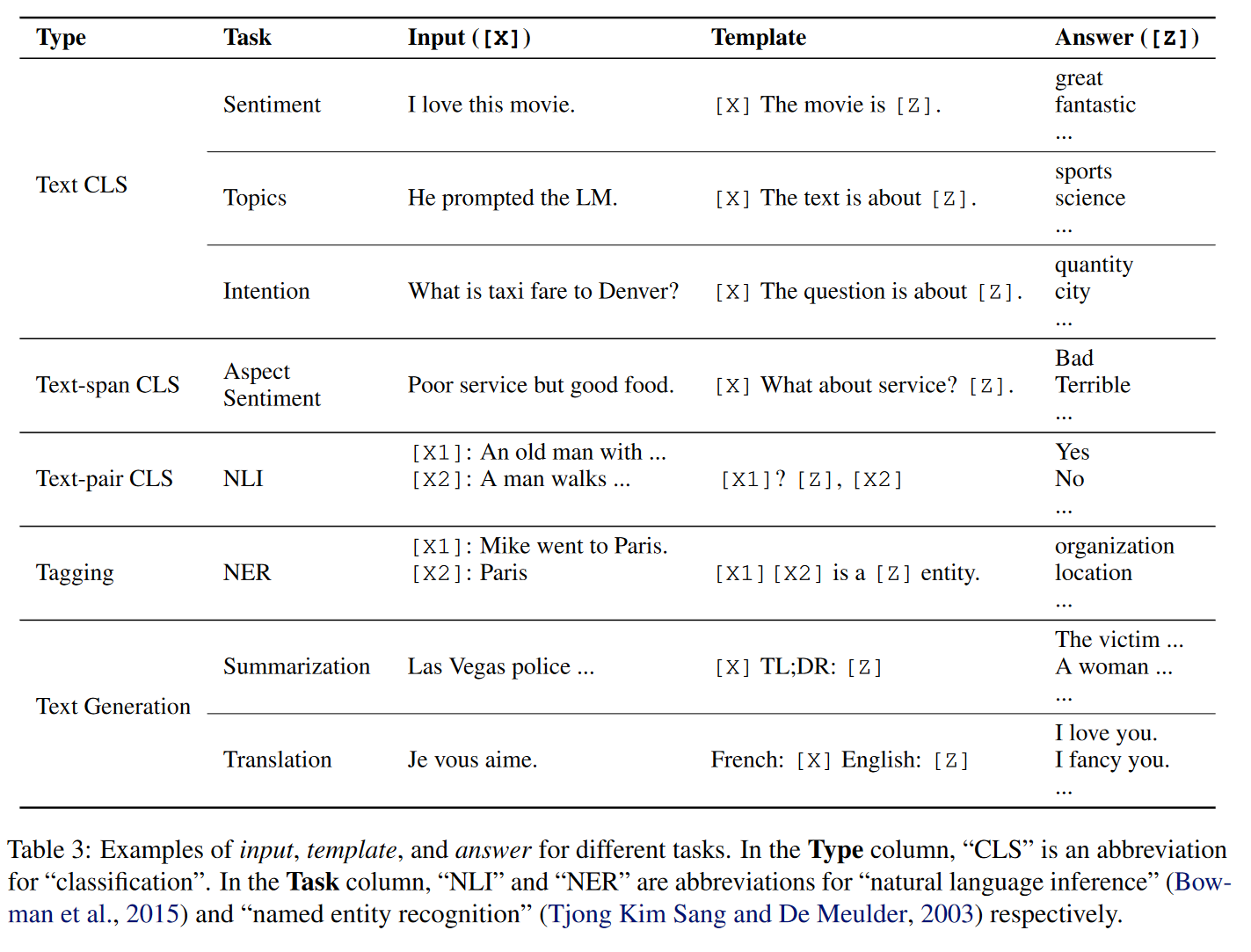## 3. Prompt Tuning

### 3.1 Fine-tune 的策略

• Linear: 只微调分类器 (一个线性层), 冻结整个骨干网络.
• Partial-k: 只微调骨干网络最后的 k 层, 冻结其他层2 3.
• MLP-k: 增加一个 k 层的 MLP 作为分类器.
• Side-tuning4: 训练一个 “side” 网络, 然后融合预训练特征和 “site” 网络的特征后输入分类器.
• Bias: 只微调预训练网络的 bias 参数5 6.
• Adapter7: 通过残差结构, 把额外的 MLP 模块插入 Transformer.

Transformer 与 ConvNet 比较

Transformer 相比于 ConvNet 的一个显著的特点是: 它们在对于空间(时间)维度的操作是不同的.

• ConvNet: 卷积核在空间维度上执行卷积操作, 因此空间内不同位置的特征通过卷积(可学习的)操作融合信息, 且只在局部区域融合.
• Transformer: 空间(时间)维度内不同位置的特征通过 Attention (非学习的) 操作融合信息, 且在全局上融合.

Transformer 在特征融合时非学习的策略使得其很容易的通过增加额外的 feature 来扩展模型.

### 3.2 NLP 中 基于 Prompt 的 fine-tune

• Prefix-Tuning
• Prompt-Tuning
• P-Tuning
• P-Tuning-v2

### 3.3 CV 中 基于 Prompt 的 fine-tuning

#### 3.3.1 分类

Visual Prompt Tuning8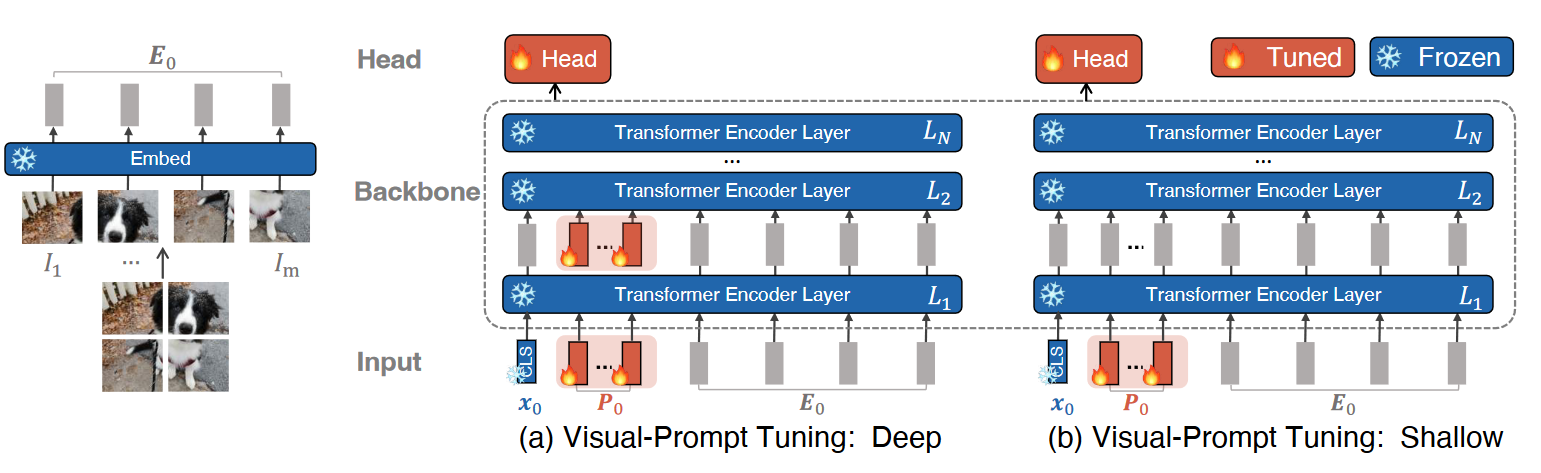• VPT-Shallow
\begin{align} [\mathbf{x}_1, \mathbf{Z}_1, \mathbf{E}_1] &= \textcolor{RoyalBlue}{L_1}([\mathbf{x}_0, \textcolor{Red}{\mathbf{P}}, \mathbf{E}_0]) \\ [\mathbf{x}_i, \mathbf{Z}_i, \mathbf{E}_i] &= \textcolor{RoyalBlue}{L_i}([\mathbf{x}_{i-1}, \mathbf{Z}_{i-1}, \mathbf{E}_{i-1}]) \qquad i=2,3,\dots,N \\ y &= \textcolor{Red}{\text{Head}}(x_N) \\ \end{align}
• VPT-Deep
\begin{align} [\mathbf{x}_i, \_, \mathbf{E}_i] &= \textcolor{RoyalBlue}{L_i}([\mathbf{x}_{i-1}, \textcolor{Red}{\mathbf{P}_{i-1}}, \mathbf{E}_{i-1}]) \qquad i=1,2,\dots,N \\ y &= \textcolor{Red}{\text{Head}}(x_N) \end{align}#### 3.3.2 持续学习

Learning to Prompt for Continue Learning9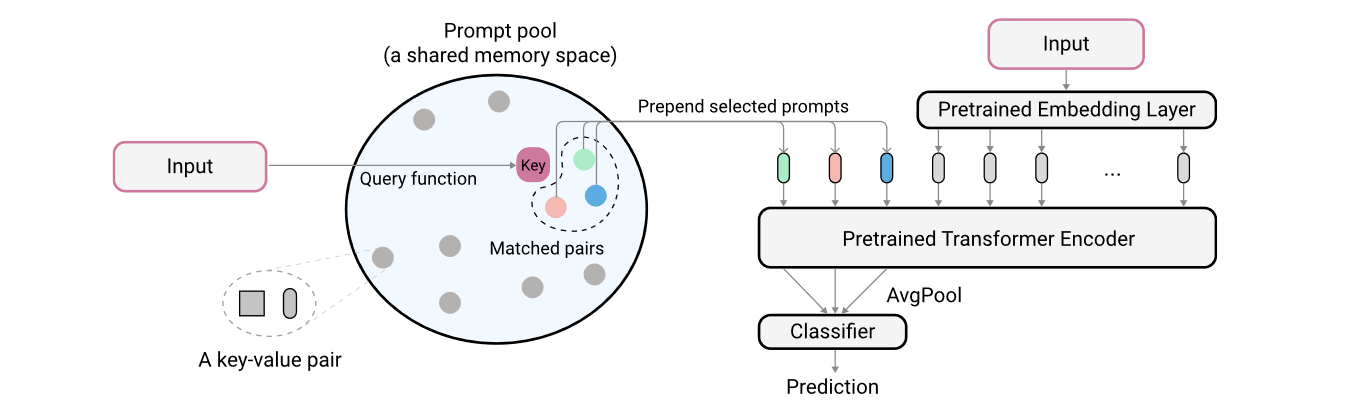$\text{min}_{\mathbf{P, K}, \phi} \mathcal{L}(g_{\phi}(f_r^{avg}(\mathbf{x}_p)), y) + \lambda\sum_{\mathbf{K}_{\mathbf{x}}}\gamma(q(\mathbf{x}), \mathbf{k}_{s_i})$

#### 3.3.3 多模态模型

Vision-Language Model: Context Optimization (CoOp)10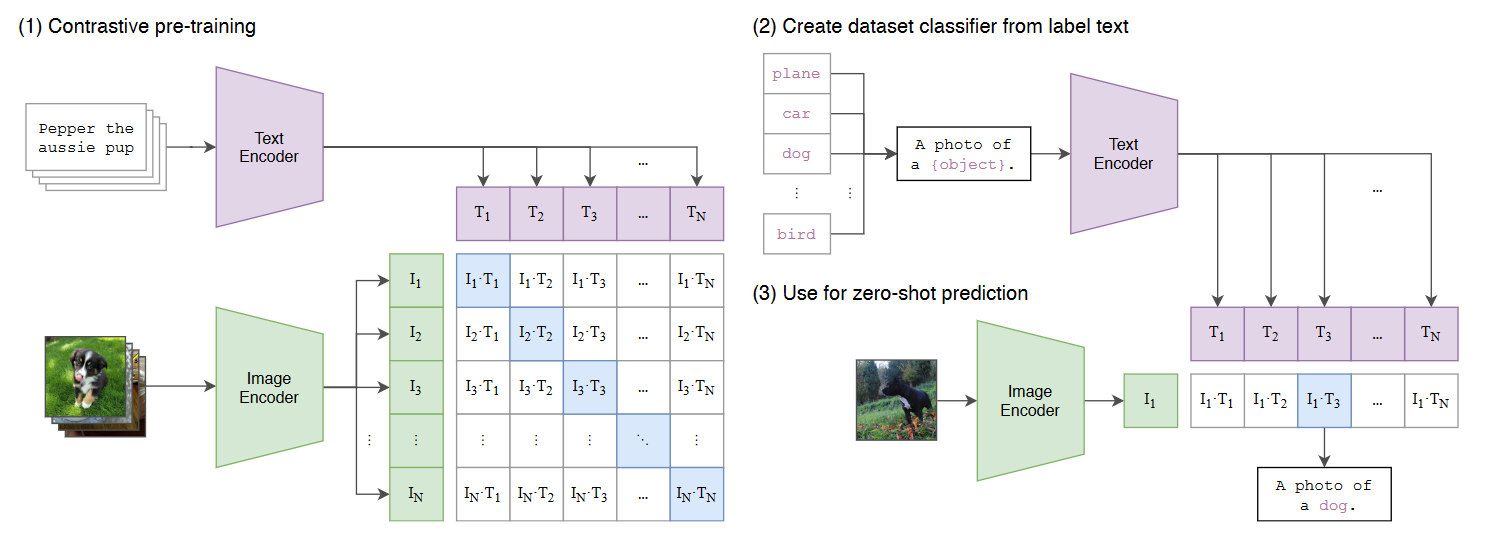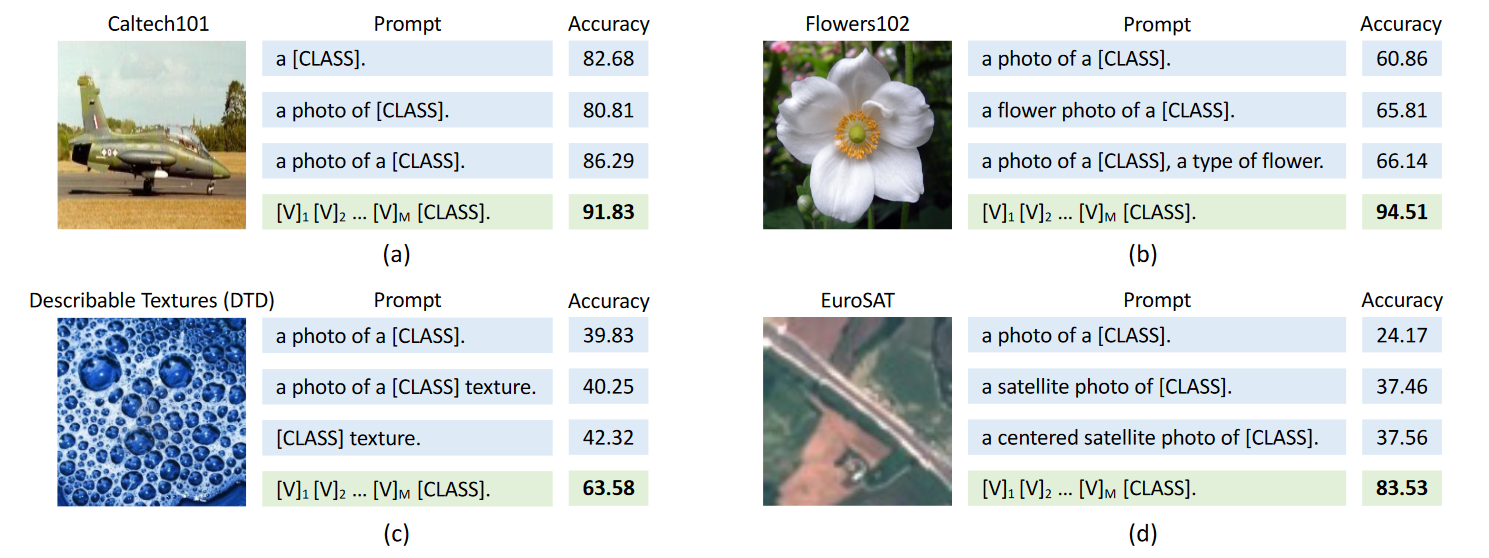• [CLASS] 放在后面: $$t = [\text{V}]_1[\text{V}]_2\dots[\text{V}]_M[\text{CLASS}]$$
• [CLASS] 放在中间: $$t = [\text{V}]_1\dots[\text{V}]_{\frac{M}{2}}[\text{CLASS}][\text{V}]_{\frac{M}{2}+1}\dots[\text{V}]_M$$

Prompt 可以在不同类之间公用, 也可以为每个类使用不同的 prompts (对于细粒度分类任务更有效).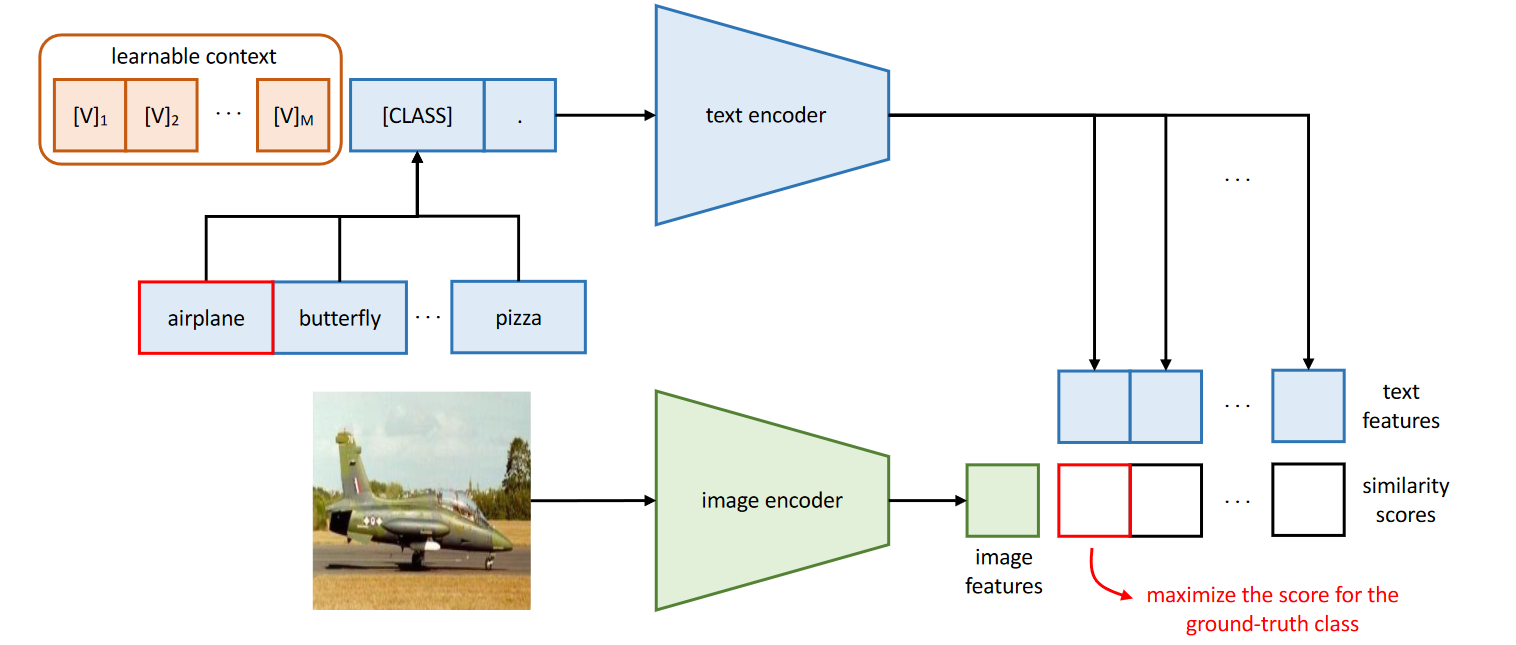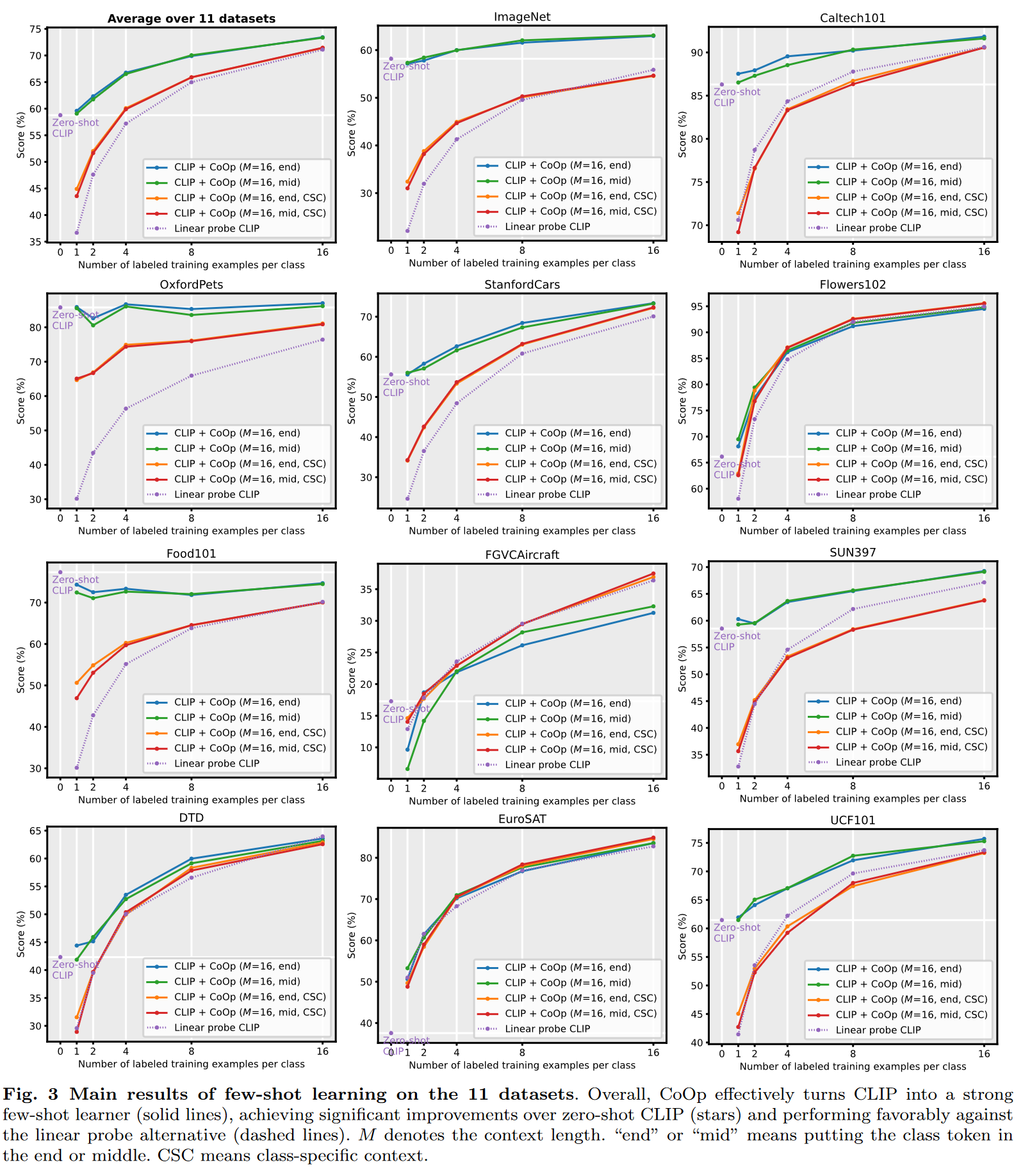Conditional Prompt Learning for Vision-Language Models11

CoOp 在泛化到新的类别上时性能不好.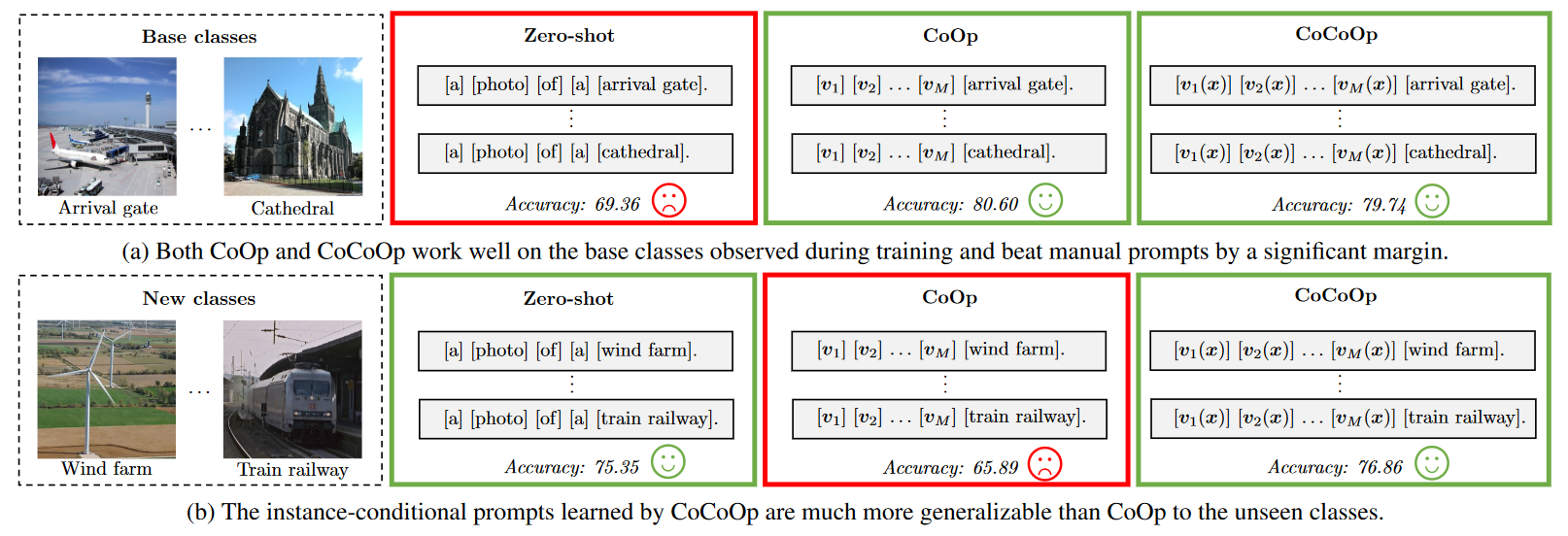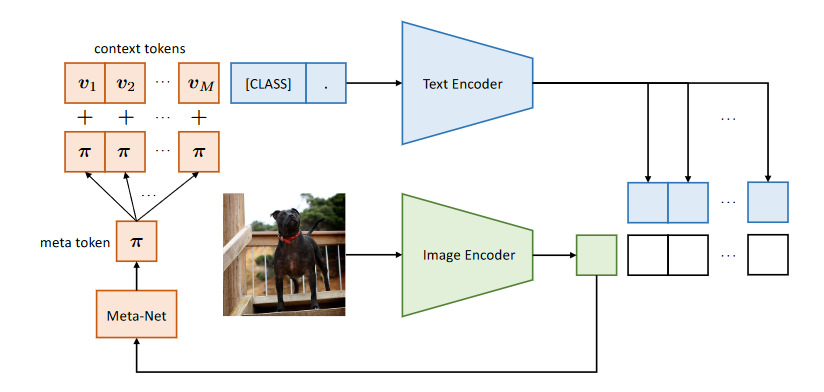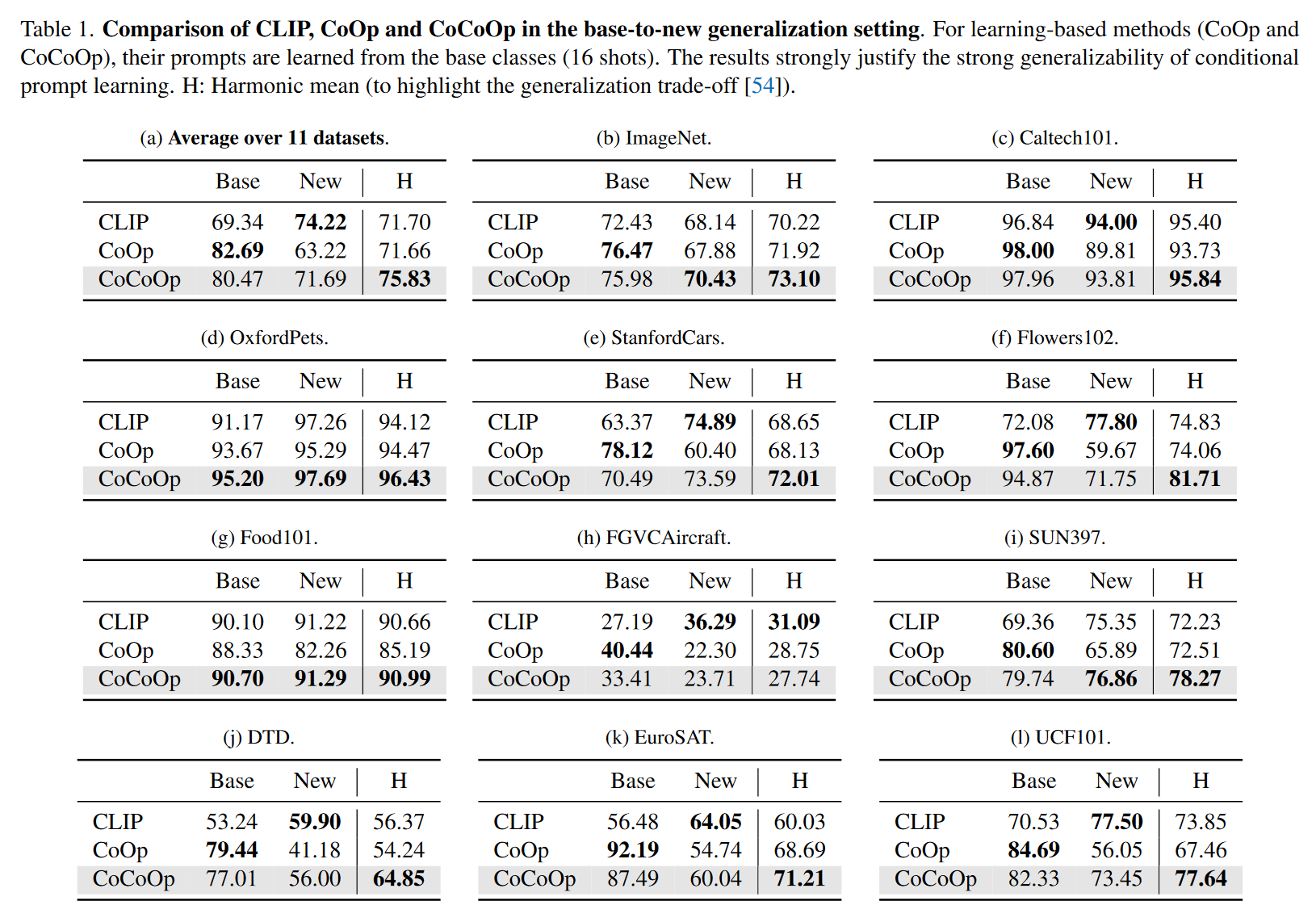#### 3.3.4 域适应$P(\hat{y}_i^s=k|\mathbf{x}_i^s) = \frac{\exp(\langle g(\mathbf{t}_k^s), f(\mathbf{x}_i^s)\rangle/T)}{\sum_{d\in\{s,u\}}\sum_{j=1}^K\exp(\langle g(\mathbf{t}_j^s), f(\mathbf{x}_i^s)\rangle/T)}$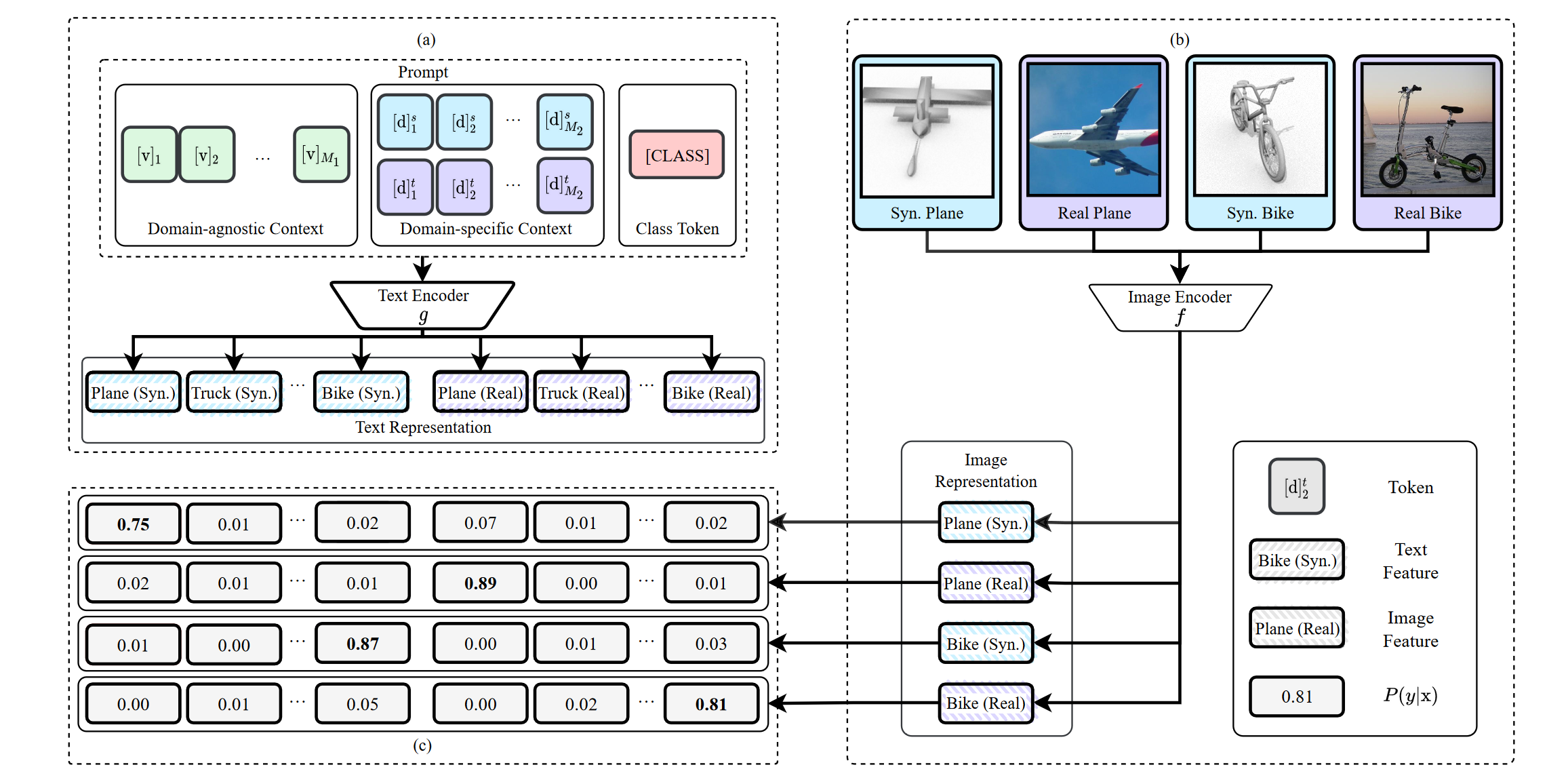## Reference

1. Pre-train, Prompt, and Predict: A Systematic Survey of Prompting Methods in Natural Language Processing
Pengfei Liu, Weizhe Yuan, Jinlan Fu, Zhengbao Jiang, Hiroaki Hayashi, Graham Neubig
[html] In arXiv 2021

2. How transferable are features in deep neural networks?
Jason Yosinski, Jeff Clune, Yoshua Bengio, Hod Lipson
[html] In NeruIPS 2014

3. Masked autoencoders are scalable vision learners
Kaiming He, Xinlei Chen, Saining Xie, Yanghao Li, Piotr Dollár, Ross Girshick
[html] In arXiv 2021

Jeffrey O. Zhang, Alexander Sax, Amir Zamir, Leonidas Guibas, Jitendra Malik
[html] In ECCV 2020

5. Bitfit: Simple parameter-efficient fine-tuning for transformer-based masked language-models.
Elad Ben Zaken, Shauli Ravfogel, Yoav Goldberg
[html] In ACL 2022

6. TinyTL: Reduce memory, not parameters for efficient on-device learning
Han Cai, Chuang Gan, Ligeng Zhu, Song Han
[html] In NeurIPS 2020

7. Parameter-efficient transfer learning for nlp
Neil Houlsby, Andrei Giurgiu, Stanislaw Jastrzebski, Bruna Morrone, Quentin De Laroussilhe, Andrea Gesmundo, Mona Attariyan, Sylvain Gelly
[html] In ICML 2019

8. Visual Prompt Tuning
Menglin Jia, Luming Tang, Bor-Chun Chen, Claire Cardie, Serge Belongie, Bharath Hariharan, Ser-Nam Lim
[html] In arXiv 2022

9. Learning to Prompt for Continual Learning
Zifeng Wang, Zizhao Zhang, Chen-Yu Lee, Han Zhang, Ruoxi Sun, Xiaoqi Ren, Guolong Su, Vincent Perot, Jennifer Dy, Tomas Pfister
[html] In CVPR 2022

10. Learning to Prompt for Vision-Language Models
Kaiyang Zhou, Jingkang Yang, Chen Change Loy, Ziwei Liu
[html] In arXiv 2021

11. Conditional Prompt Learning for Vision-Language Models
Kaiyang Zhou, Jingkang Yang, Chen Change Loy, Ziwei Liu
[html] In CVPR 2022

12. Domain Adaptation via Prompt Learning
Chunjiang Ge, Rui Huang, Mixue Xie, Zihang Lai, Shiji Song, Shuang Li, Gao Huang
[html] In arXiv 2022

Content